# A five-digit

A five-digit odd number has the sum of all digits (digits) five and contains two zeros. If we move each digit in the number one place to the left and move the first digit to the last place, we get a number 20,988 smaller. Find this unknown five-digit number.

x =  31001

### Step-by-step explanation: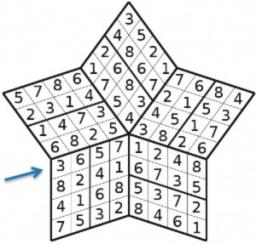Did you find an error or inaccuracy? Feel free to write us. Thank you!## Related math problems and questions:

• Number train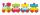The numbers 1,2,3,4,5,6,7,8 and 9 traveled by train. The train had three cars and each was carrying just three numbers. No. 1 rode in the first carriage, and in the last carriage was all odd numbers. The conductor calculated sum of the numbers in the firs
• Four-digit numbers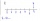Find four-digit numbers where all the digits are different. For numbers, the sum of the third and fourth digits is twice the sum of the first two digits, and the sum of the first and fourth digits is equal to the sum of the second and third digits. The di
• Dd 2-digit numbersFind all odd 2-digit natural numbers compiled from digits 1; 3; 4; 6; 8 if the digits are not repeated.
• Unknown xIf we add to unknown number 21, then divide by 6 and then subtract 51, we get back an unknown number. What is this unknown number?
• Number with onesThe first digit of the number is 1, if we move this digit to the end we get a 3 times higher number, which is the number?
• Perpetrator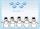The perpetrator is a number that is smaller than the number 80. It is a multiple of five and it is odd. If we added up the tens and ones of this number we get number 8.
• Street numbersLada came to aunt. On the way he noticed that the houses on the left side of the street have odd numbers on the right side and even numbers. The street where he lives aunt, there are 5 houses with an even number, which contains at least one digit number 6
• Three numbersWe have three different non-zero digits. We will create all 3 digits numbers from them to use all 3 figures in each number. We add all the created numbers, and we get the sum of 1554. What were the numbers?
• Sum of the digitsHow many are two-digit natural numbers that have the sum of the digits 9?
• Last digitWhat is the last number of 2016 power of 2017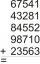Fill letters instead of digits so the indicated sum (equal letters represent equal digits). What number is hidden under the letter J? A A H A H O A H O J -------------------------- 4 3 2 1In a two-digit number, the number of tens is three greater than the number of units. If we multiply the original number by a number written in the same digits but in reverse order, we get product 3 478. Find the original number.I know two numbers whose sum is 20. When they each round and then added together I get the 30. What are this numbers?Find the largest integer such that: 1. No figures is not repeat, 2. multiplication of every two digits is odd, 3. addition all digits is odd.From the digits 1,2,3,4 we create the long integer number 123412341234. .. .. , which will have 962 digits. Is this number divisible by 6?How many odd four-digit numbers can we create from digits: 0, 3, 5, 6, 7? (a) the figures may be repeated (b) the digits may not be repeatedFind the sum of all odd integers from 13 to 781.